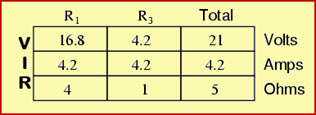# A Brief on Thevenins Theorem with Examples

Engineering in electrical and electronics stream is comprised of several engineering subjects that includes basic topics such as laws like Ohm’s law, Kirchoff’s law, etc., and network theorems These laws and theorems are used for solving the complex electrical circuits and mathematical calculations to find out network parameters such as current, voltage, and so on in electrical network analysis. These network theorems include thevenins theorem, Norton’s theorem, Reciprocity theorem, Superposition theorem, Substitution theorem, and Maximum power transfer theorem. Here, in this article let us discuss in detail about how to state thevenins theorem, thevenins theorem examples, and applications of thevenins theorem.

## Thevenins Theorem

Network theorem that is used for reducing a large, complex linear electric circuit that consists of several voltages or/and current sources and several resistances into a small, simple electric circuit with one voltage source with one series resistance connected across it is termed as thevenins theorem. The thevenins theorem statement helps us to better understand about thevenins theorem very easily in a single sentence.

### Thevenins Theorem Statement

Thevenins theorem states that any linear electrically complex circuit is reduced into a simple electric circuit with one voltage and resistance connected in series. For understanding in depth regarding thevenins theorem let us consider thevenins theorem examples as follows.

#### Thevenins Theorem Examples

Primarily, consider a simple example circuit with two voltage sources and three resistors which are connected to form an electrical network as shown in the figure below.

In the above circuit, the V1=28V, V2=7V are two voltage sources and R1=4 Ohm, R2=2 Ohm, and R3=1 Ohm are three resistances among which let us consider the R2 resistor as load resistance. As we know that, based on the load conditions the load resistance is accordingly varied and thus, the total resistance has to be calculated based on how many resistors are connected in the circuit which is very critical.

So, to make it easier thevenins theorem states that the load resistor has to be removed temporarily and then calculate the circuit voltage and resistance by reducing it to a single voltage source with a single series resistor. Thus, the equivalent circuit formed is termed as thevenins equivalent circuit (as shown in the above figure) having equivalent voltage source called as thevenins voltage and equivalent resistor called as thevenins resistance.

Then, the equivalent thevenins circuit can be represented as shown in the above figure. Here, in this circuit is equivalent to the above circuit (with V1, V2, R1, R2, and R3) in which the load resistance R2 is connected across the terminals of thevenins equivalent circuit as shown in the circuit below.

Now, how to find out the values of thevenins voltage and thevenins resistance? For this, we must apply basic rules (based on a series or parallel circuit which is formed after removing load resistance) and also by following the principles of Ohm’s law and Krichhoff’s law.

Here, in this example the circuit formed after removing load resistance is series circuit. Hence, the thevenins voltage or voltage across the load resistance terminals which is open circuited can be determined using above mentioned laws (Ohm’s law and Krichhoff’s law) and are tabulated in a tabular form as shown below:Then, the circuit can be represented as shown in the figure below with voltage across open load terminals, resistances, and current in the circuit. This voltage across the open load resistance terminals is termed as thevenins voltage which is to be placed in the thevenins equivalent circuit.

Now, the thevenins equivalent circuit with load resistance connected in series with the thevenins voltage and thevenins resistance as shown in the figure below.

To find out the thevenins resistance, the original circuit has to be considered and the load resistance has to be removed. In this circuit, similar to superposition principle, i.e., open circuit the current sources and short circuit voltage sources in the circuit. Thus, the circuit becomes as shown in the figure below in which the resistances R1 and R3 are parallel to each other.